## A manufacturer of potato chips would like to know whether its bag filling machine works correctly at the 443 gram setting. It is believed th

Question

A manufacturer of potato chips would like to know whether its bag filling machine works correctly at the 443 gram setting. It is believed that the machine is underfilling the bags. A 15 bag sample had a mean of 434 grams with a standard deviation of 17. A level of significance of 0.1 will be used. Assume the population distribution is approximately normal. Determine the decision rule for rejecting the null hypothesis. Round your answer to three decimal places.

in progress 0
5 months 2021-09-05T10:15:38+00:00 1 Answers 2 views 0

Null hypothesis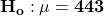Alternative hypothesis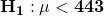t = – 2.05

Degree of freedom df = 14

P-value = 0.0298

Decision rule: To reject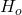if significance level ∝  is greater than P-value.

Conclusion: We rejectat the level of significance ∝ = 0.1, thus there is sufficient evidence to conclude that the machine is underfilling the bags.Step-by-step explanation:

Given that:

Population mean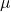= 443

Sample size n = 15

Sample mean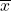= 434

standard deviation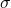= 17

Level of significance ∝ = 0.01

The null and the alternative hypothesis can be computed as:

Null hypothesisAlternative hypothesisThe t-test statistics can be computed as :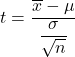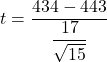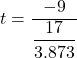t = – 2.05

Degree of freedom df = n – 1

Degree of freedom df = 15 – 1

Degree of freedom df = 14

From t distribution table; from the area in the lower tail to the left of t = -2.05 and for  the degree of freedom df = 14, it is given by 0.0298

Thus, P-value = 0.0298

Decision rule: To rejectif significance level ∝  is greater than P-value.

Conclusion: We rejectat the level of significance ∝ = 0.1, thus there is sufficient evidence to conclude that the machine is underfilling the bags.